Having trouble getting all the keys in Uncharted: Fortune Hunter? Don't worry, we've got your back. Follow these guides and you'll have them all in no time flat.

### Level 11

First move here.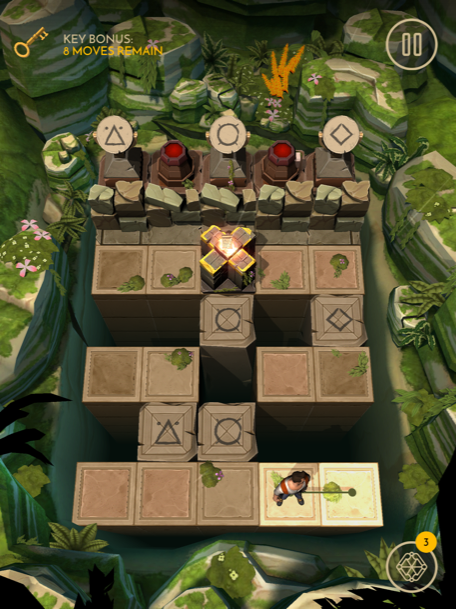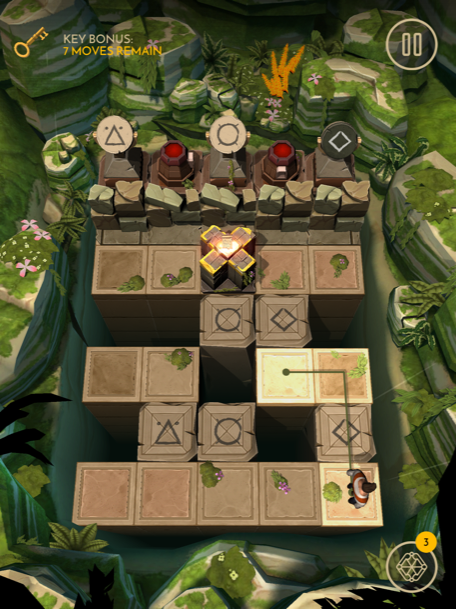Now move here.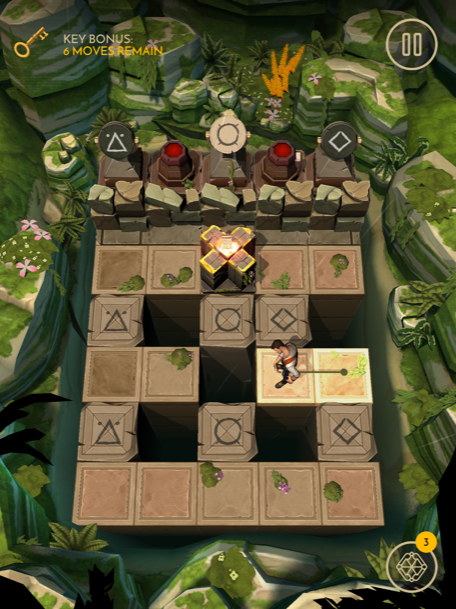Then follow this path to grab the treasure.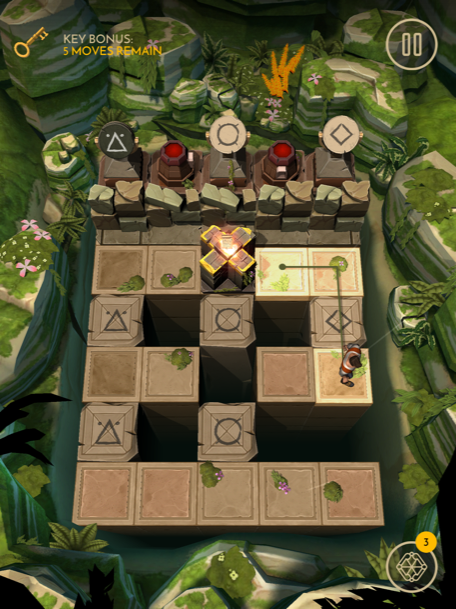### Level 12

First move here.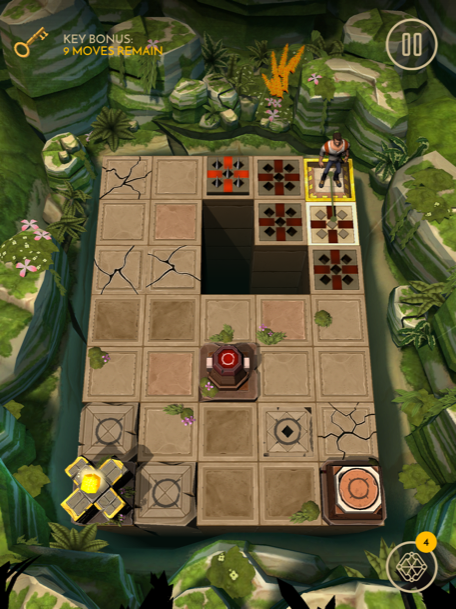Now move here.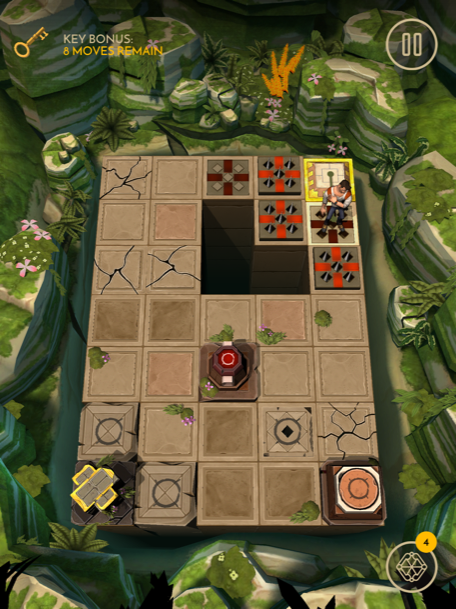Next follow this path to the switch.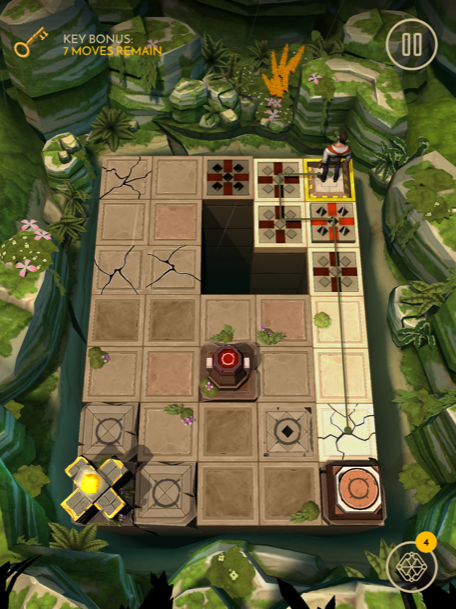Push the switch, then move here.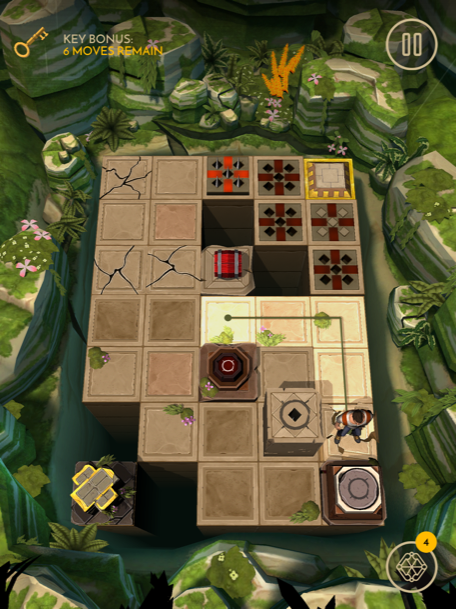Pick up the bomb, then move here.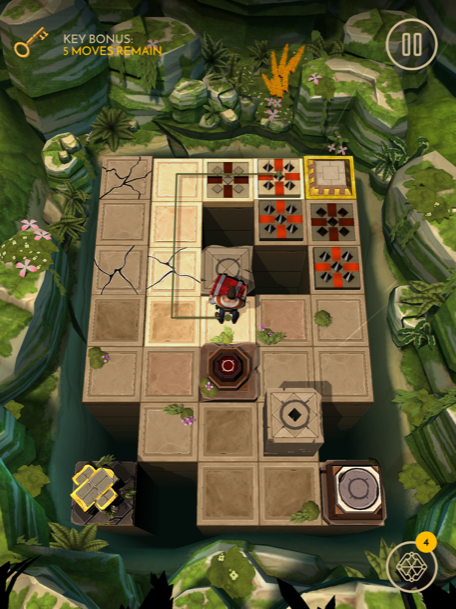Drop the bomb on the switch, then move here.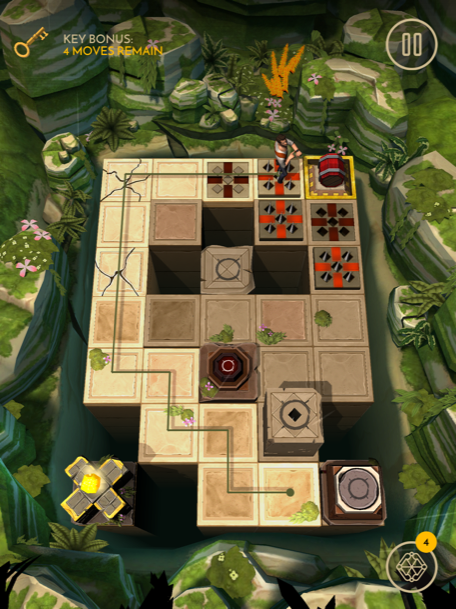Push the switch, then follow this path to grab the treasure.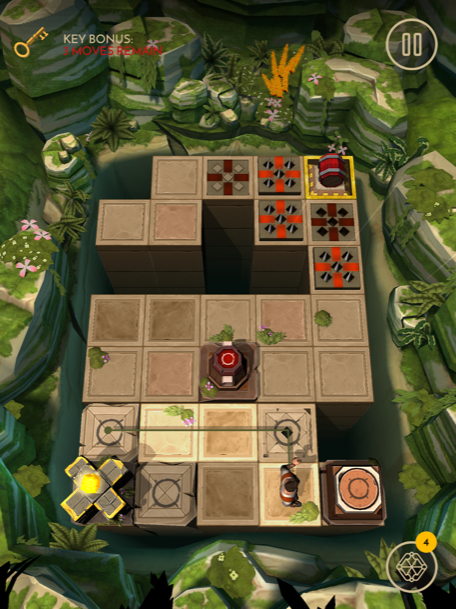### Level 13

Follow this path and grab the treasure.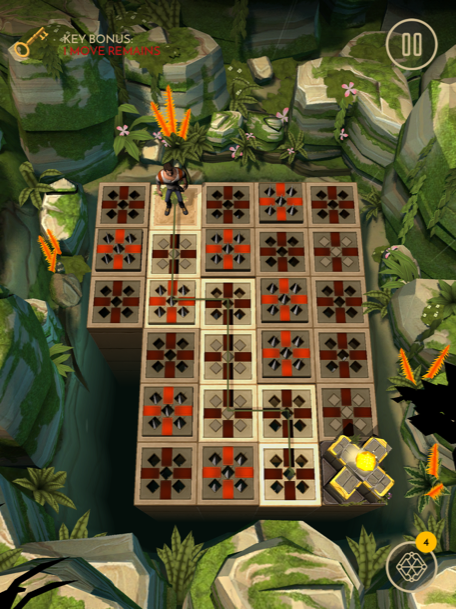### Level 14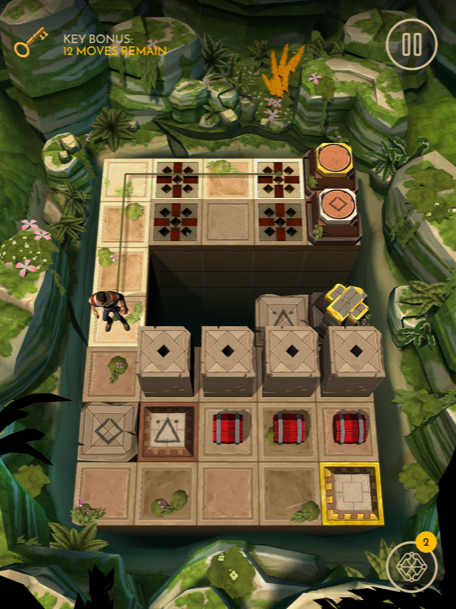Push the switch, then move here.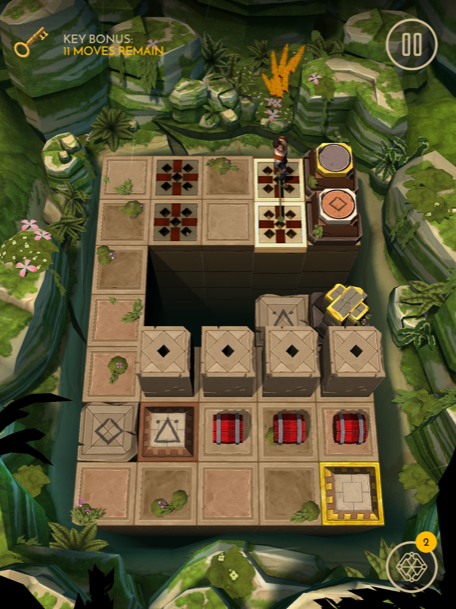Push the switch, shoot the bomb that appears, then push the switch again and move here.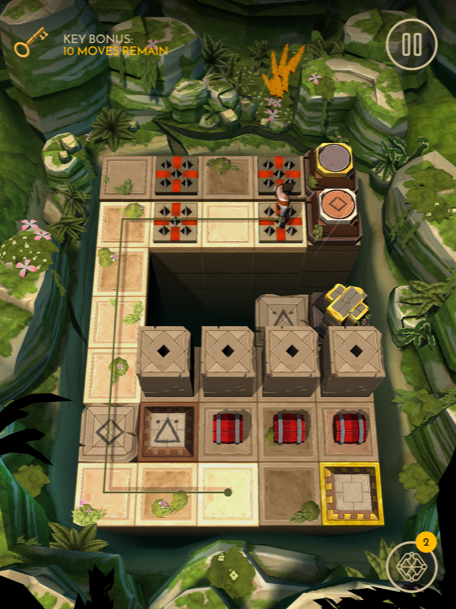Pick up the bomb then move here.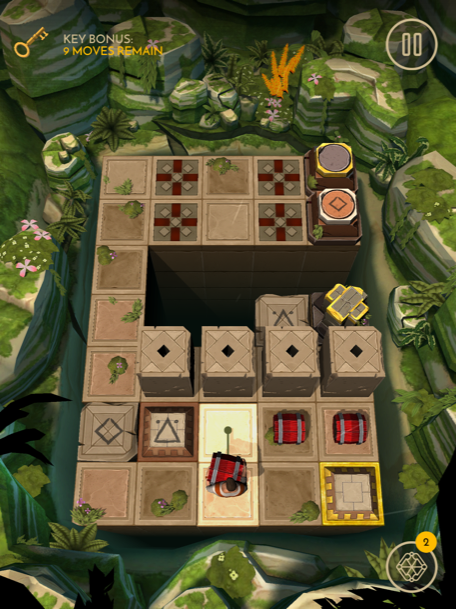Put the bomb on the switch, pick up the bomb to your right, then move here.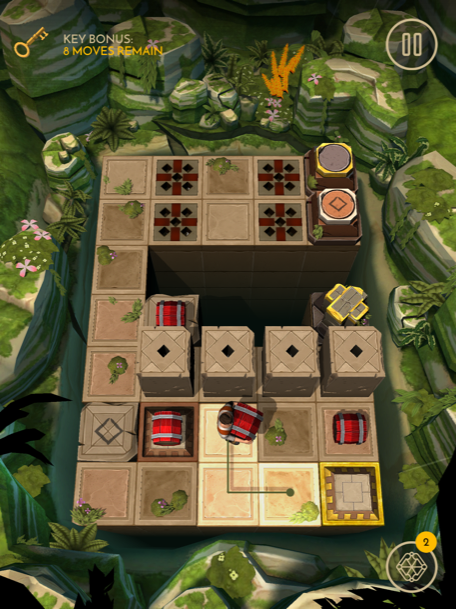Put the bomb on the switch, then move here.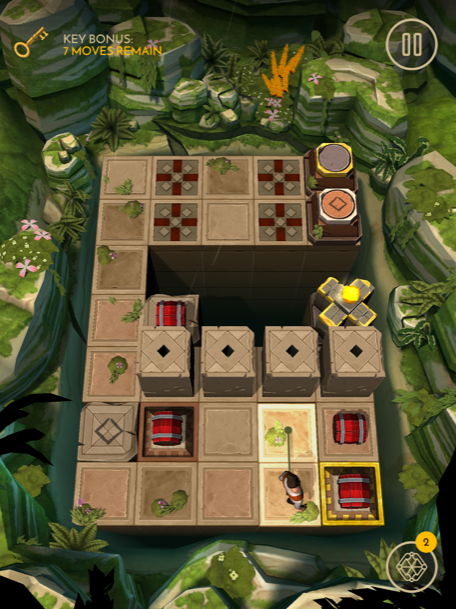Pick up the bomb, then move here.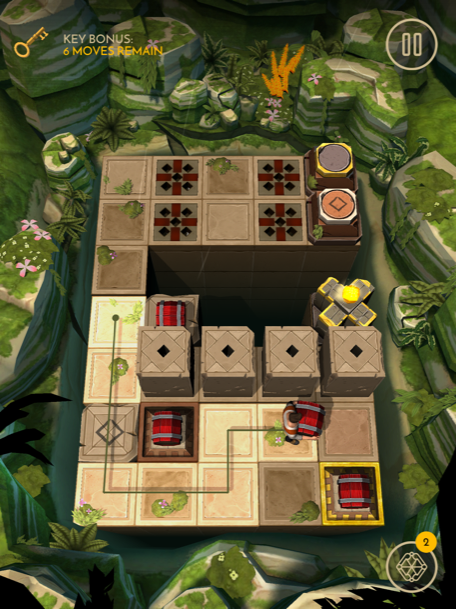Put the bomb down behind you, then pick up the bomb to your right and move here.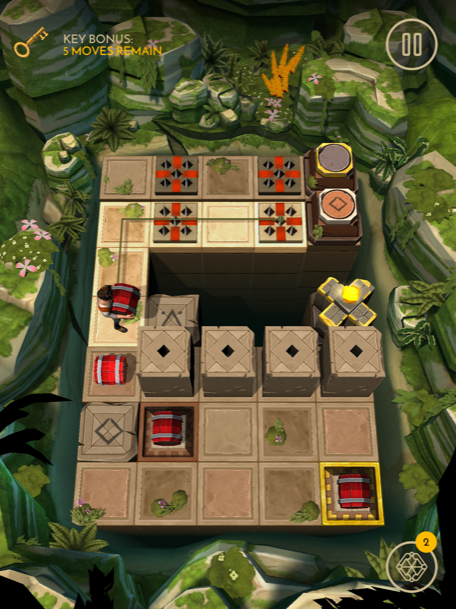Put the bomb down, push the switch, pick the bomb up again, then follow this path.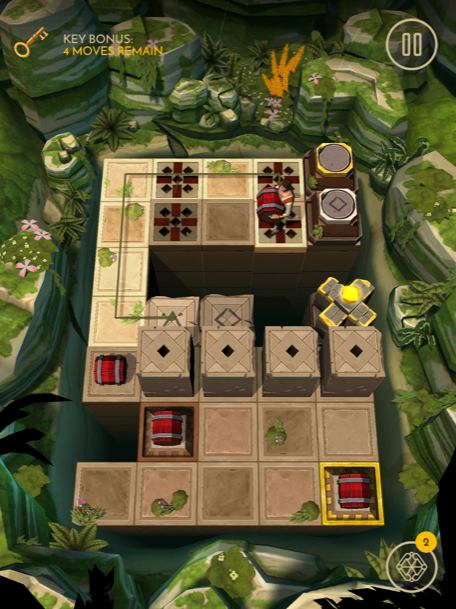Put the bomb next to the other one, then move here.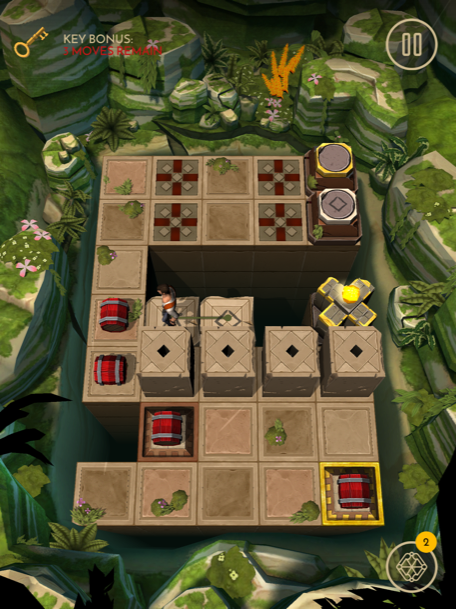Shoot the bomb, then follow this path to the treasure.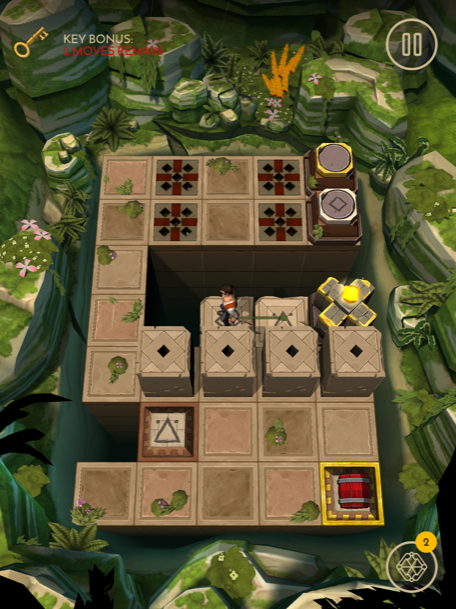### Level 15

Move here and grab the treasure.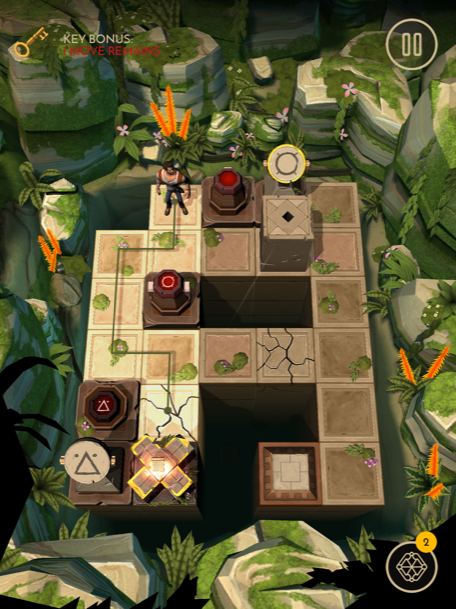### Level 16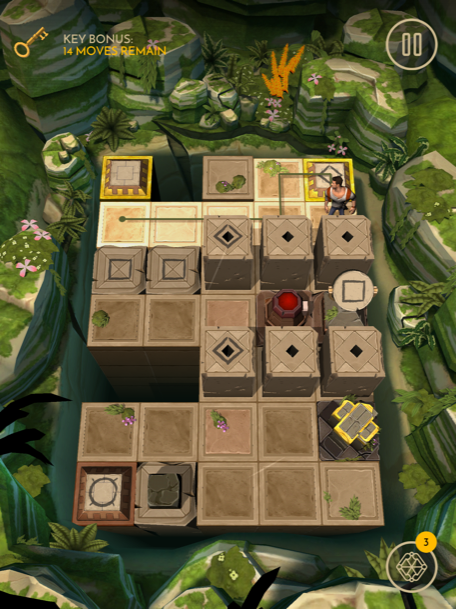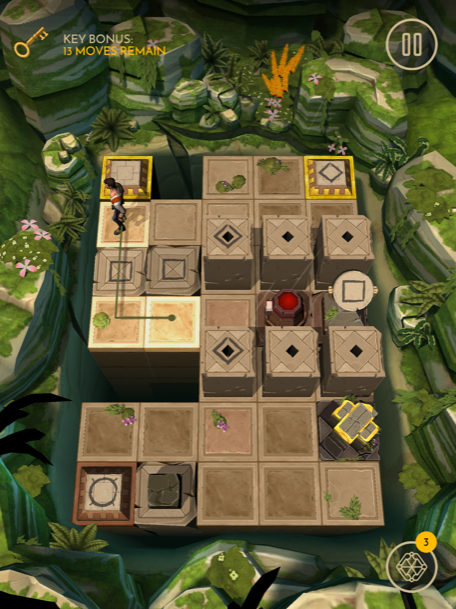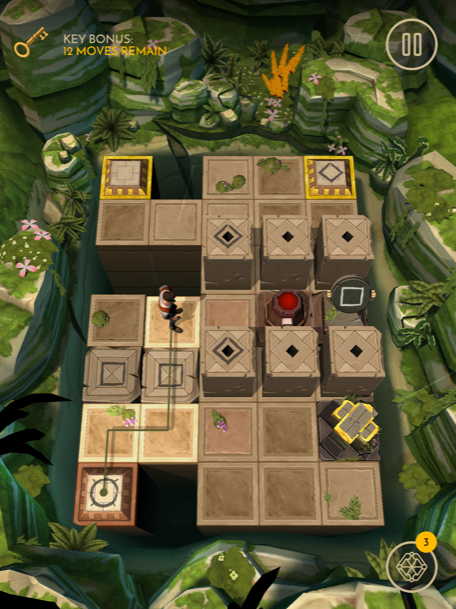Grab the block then move here.Drop the block on the switch, then move here.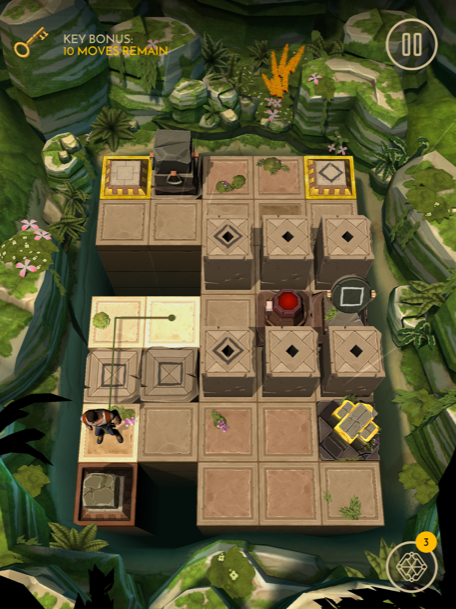Now move here.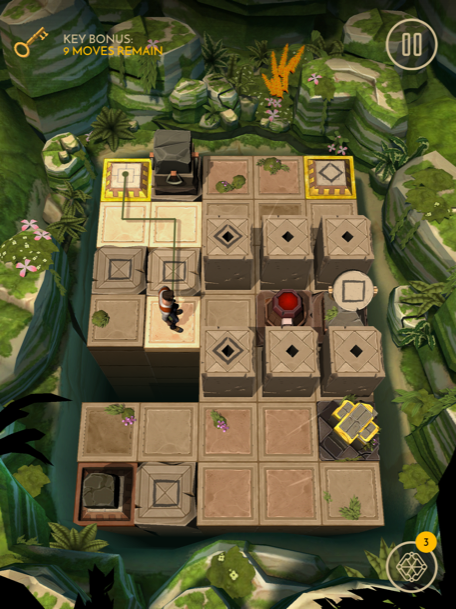Tap the block and push it here.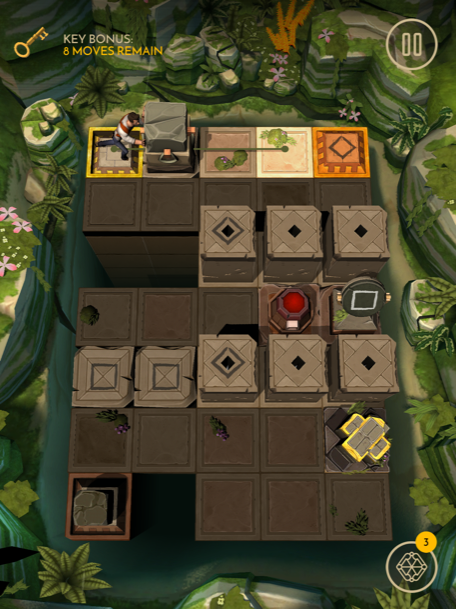Next move here and pick up the block.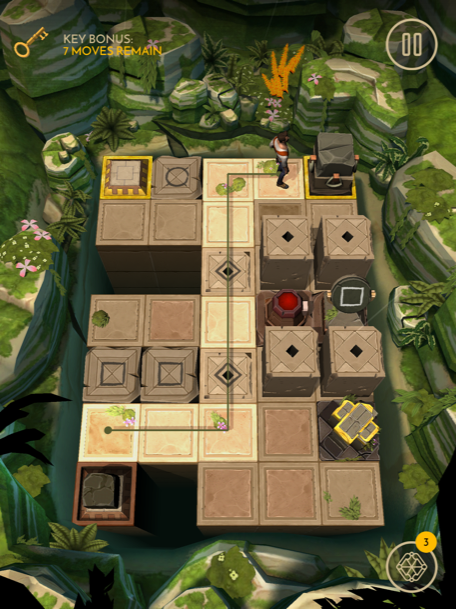Move here and drop the block on the switch.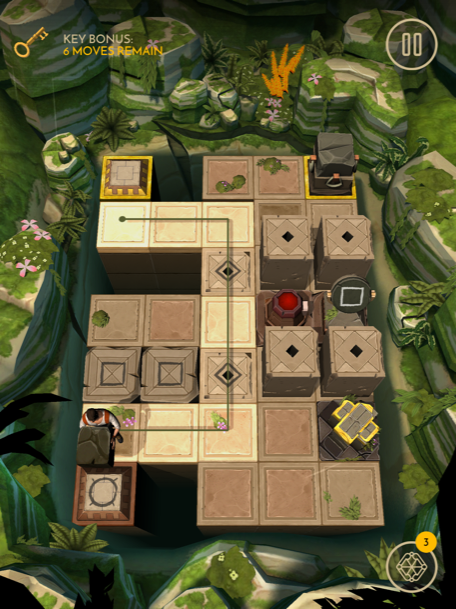Now head here and grab the treasure.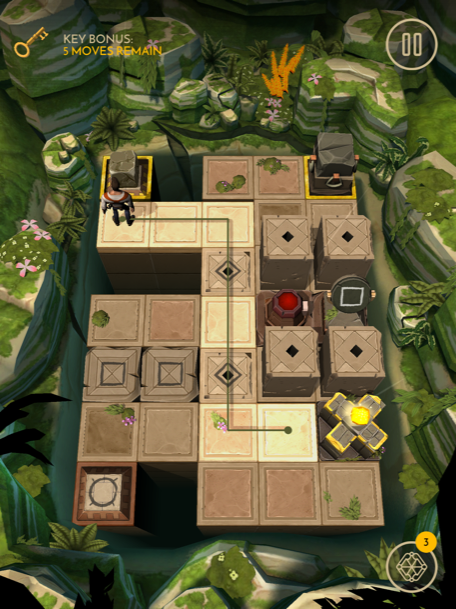### Level 17

Follow this path and grab the treasure.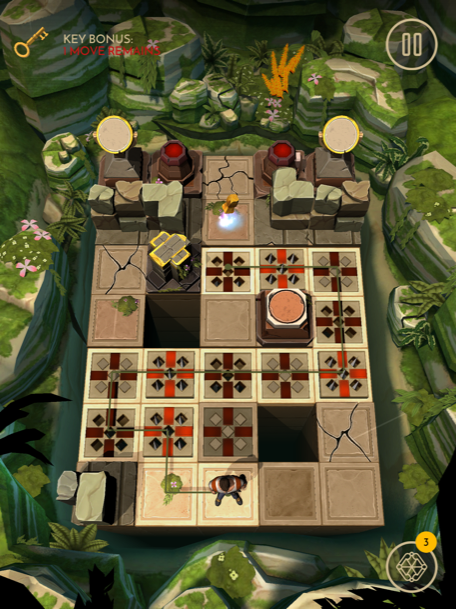### Level 18

First tap the block and push it here.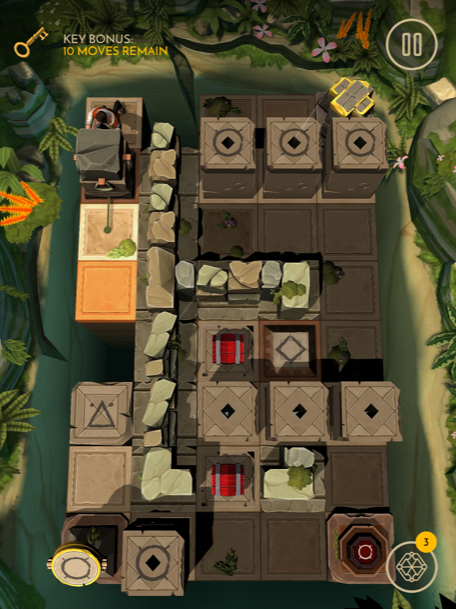Now tap the block again and push it here.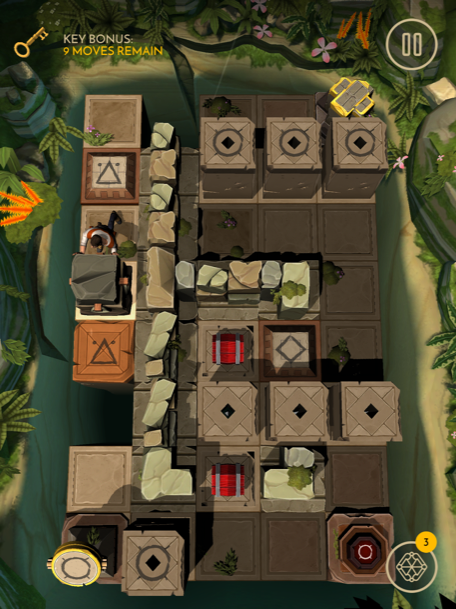Now move back here and shoot the plate.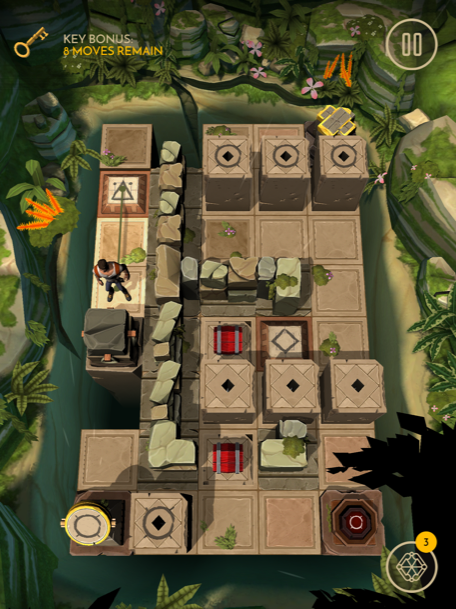Now move here.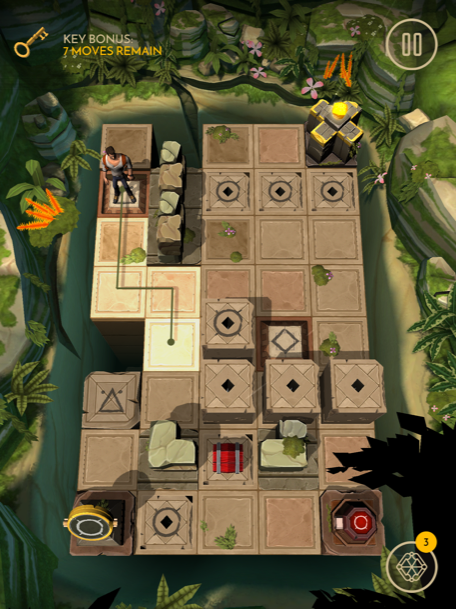Tap the block and drag it here.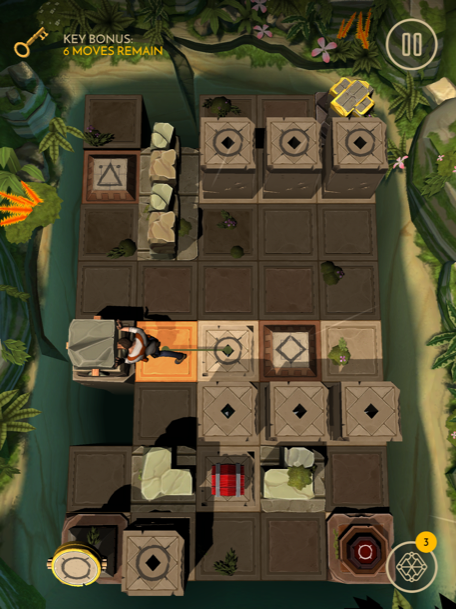Now move here.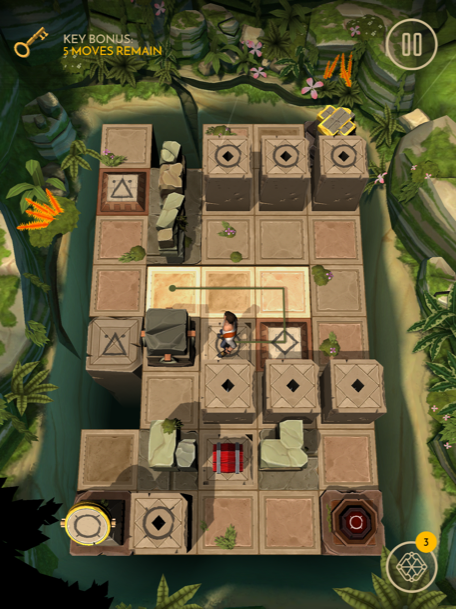Tap the block and push it here.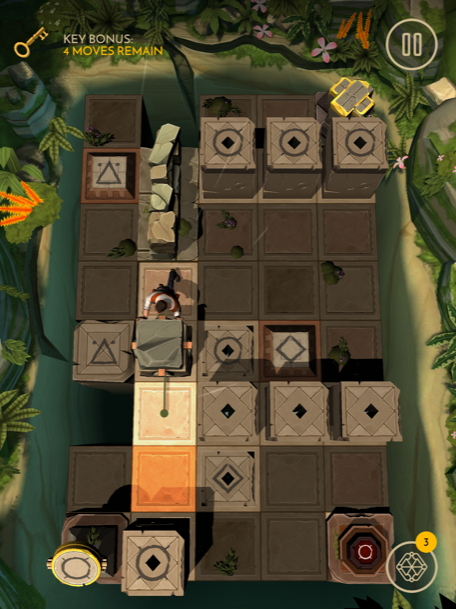Shoot the plate, tap the block, then push it here.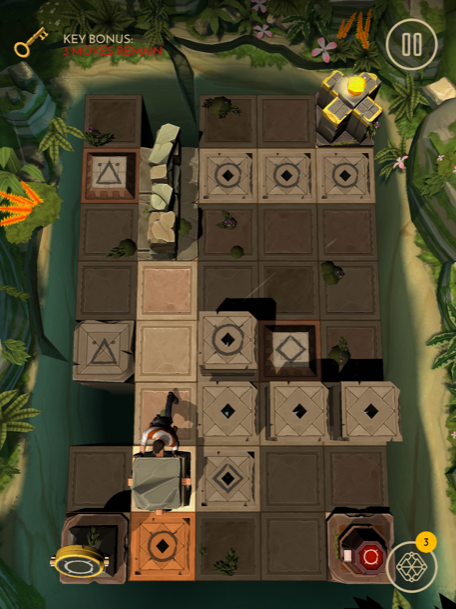Now follow this path to the treasure.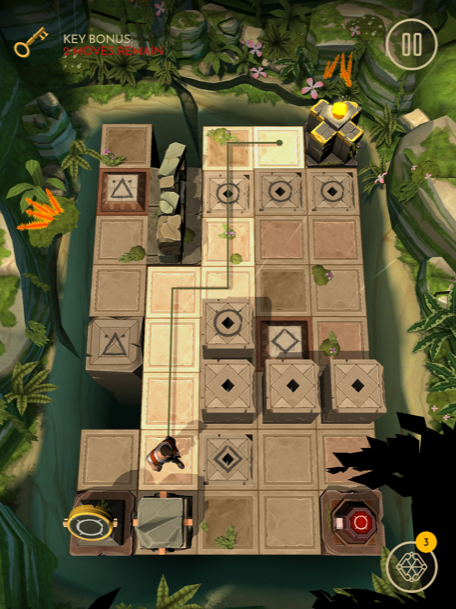### Level 19

First move here.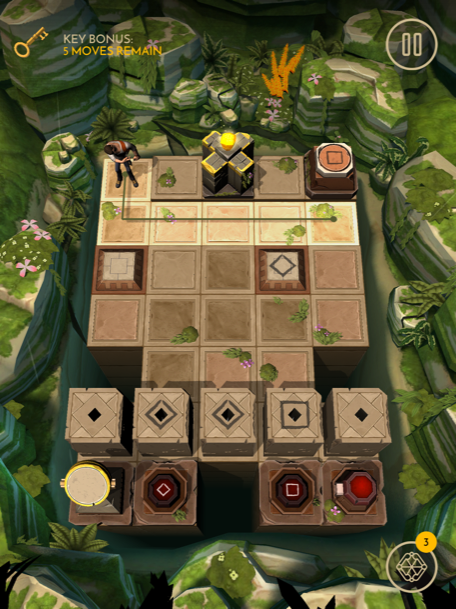Tap the button, then move here.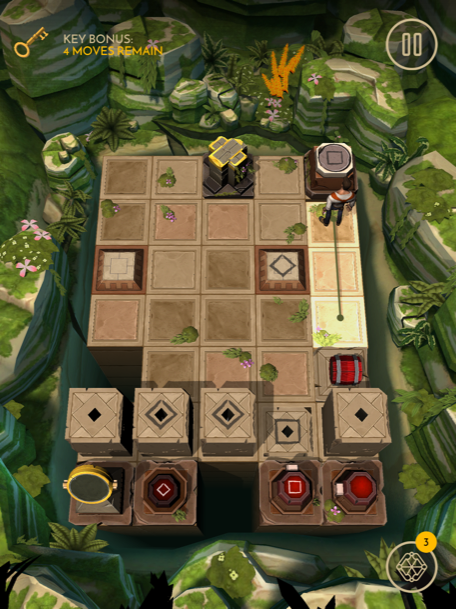Grab the bomb, then move here.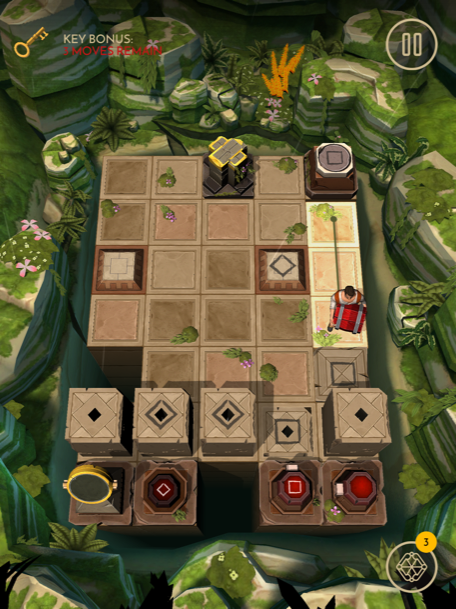Drop the bomb behind you, push the switch, pick the bomb up again, and move here.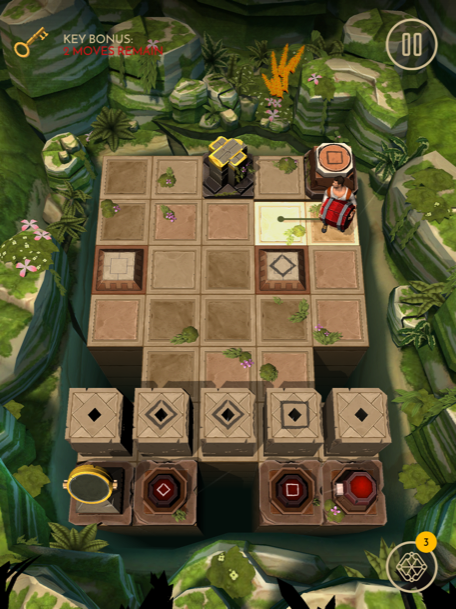Place the bomb on the switch, then move here and grab the treasure.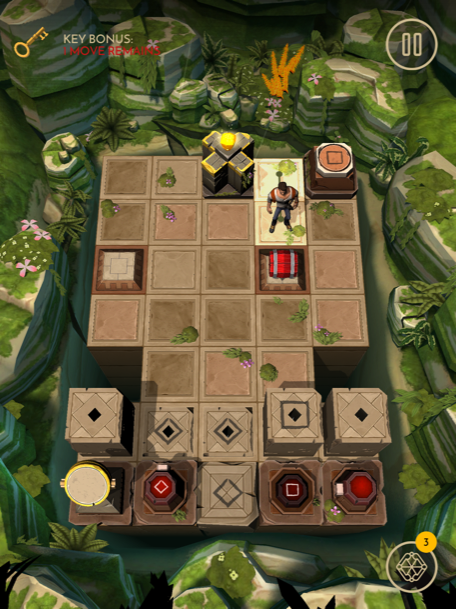### Level 20

First move here.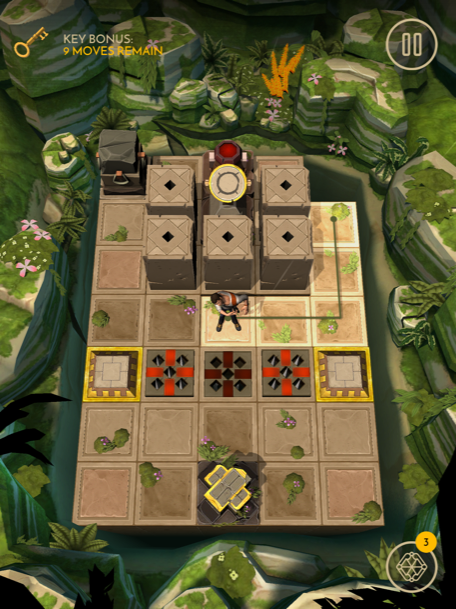Tap the block and drag it here.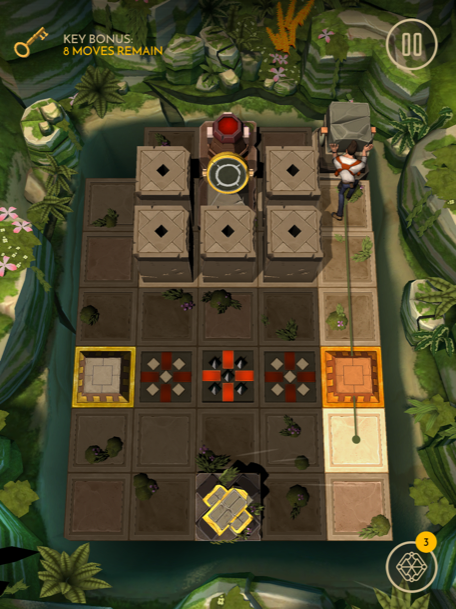Now move here.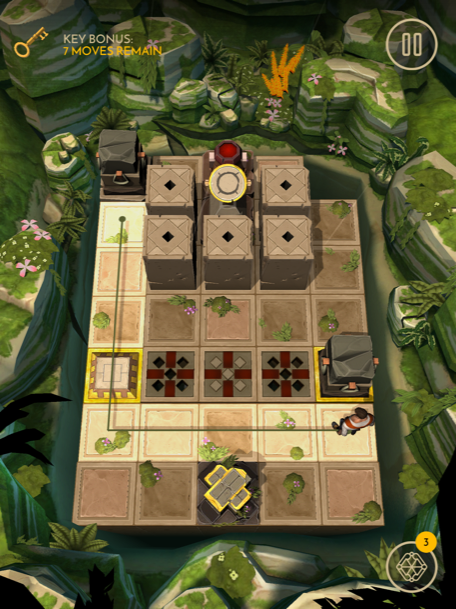Tap the block and drag it here.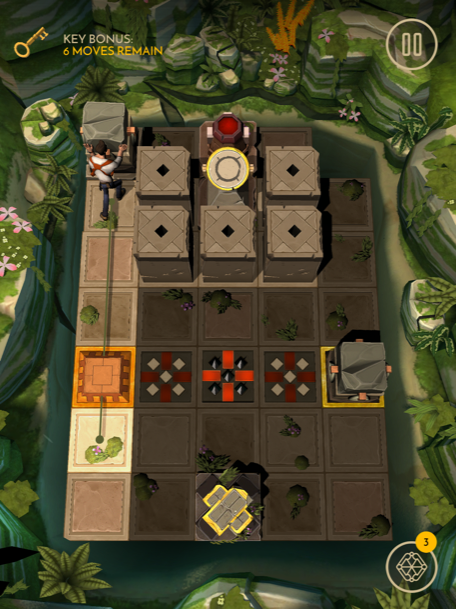Now follow this path and grab the treasure.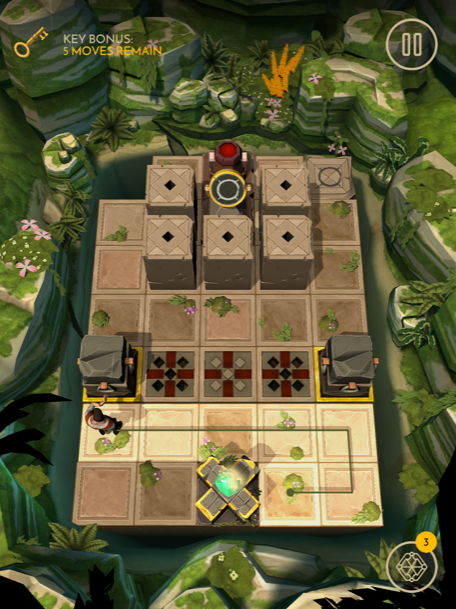Levels 1-10
Levels 21-30
Levels 31-40